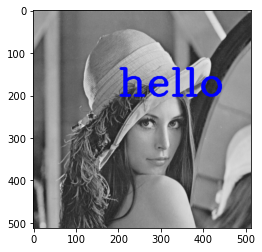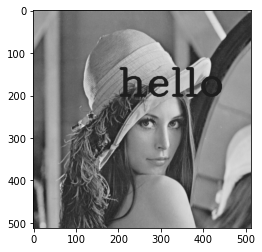## NYU CSCI-UA 9473 Introduction to ML ¶

### Unsupervised Learning (Part III)¶

#### Exercise 1. Dictionnary learning and image inpainting.¶

We consider the popular Lena image below which was corrupted by the overlaying of some text

In :
from PIL import Image

img = Image.open('lena.png').convert('LA')
img.save('greyscale.png')

In :
import cv2
import numpy as np
import matplotlib.pyplot as plt
texted_image =cv2.putText(img=np.copy(image), text="hello", org=(200,200),fontFace=3, fontScale=3, color=(0,0,255), thickness=5)
plt.imshow(texted_image)
plt.show()In :
im = Image.fromarray(texted_image)
img = im.convert('LA')
plt.imshow(img)
plt.show()Using the grayscale image above (or if you want to your implementation to be faster, on a downsampled version of this image), decompose the image into a series of overlapping patches (e.g. of size 8x8), store those patches as a list of vectors. Center the list and scale it (divide by the std). Once we have a first dictionnary, we can try to compute the recovered image by first expressing each of the patches from the original image as

\begin{align*} \underset{\mathbf{s}}{\operatorname{argmin}} \left\|\mathbf{y} - \mathbf{D}\mathbf{s}\right\| + \lambda \|\mathbf{s}\|_1 \end{align*}

This minimization can be solved for example through Matching Pursuit

In [ ]:
# implement the minimization step here


Once the decomposition of the image in the dictionnary has been found, we can the update this dictionnary as \begin{align*} \underset{\mathbf{D}}{\operatorname{argmin}} \left\|\mathbf{Y} - \mathbf{D}\mathbf{S}\right\| \end{align*} which can be solved in closed form through the steps \begin{align*} \mathbf{D} = \mathbf{Y}\mathbf{S}^T\left(\mathbf{S}\mathbf{S}^T\right)^{-1} \end{align*} followed by the normalization of the atoms (colums of the dictionnary).

In [ ]:
# add the dictionnary learning step below